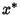## Average Function

Ifis Continuous on a Closed Interval, then there is at least one numberinsuch thatThe average value of the Functionon this interval is then given by.

See Mean-Value Theorem